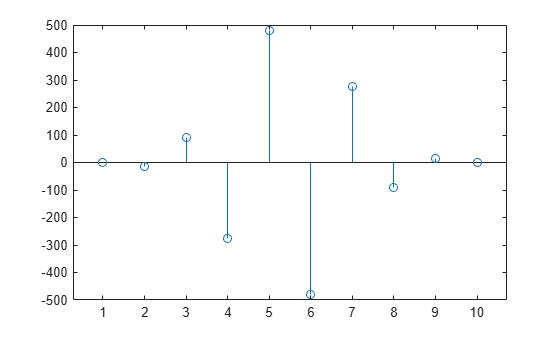Main Content

# Single Precision Math

This example shows how to perform arithmetic and linear algebra with single precision data. It also shows how the results are computed appropriately in single-precision or double-precision, depending on the input.

### Create Double Precision Data

Let's first create some data, which is double precision by default.

`Ad = [1 2 0; 2 5 -1; 4 10 -1]`
```Ad = 3×3 1 2 0 2 5 -1 4 10 -1 ```

### Convert to Single Precision

We can convert data to single precision with the `single` function.

`A = single(Ad); % or A = cast(Ad,'single');`

### Create Single Precision Zeros and Ones

We can also create single precision zeros and ones with their respective functions.

```n = 1000; Z = zeros(n,1,'single'); O = ones(n,1,'single');```

Let's look at the variables in the workspace.

`whos A Ad O Z n`
``` Name Size Bytes Class Attributes A 3x3 36 single Ad 3x3 72 double O 1000x1 4000 single Z 1000x1 4000 single n 1x1 8 double ```

We can see that some of the variables are of type `single` and that the variable `A` (the single precision version of `Ad`) takes half the number of bytes of memory to store because singles require just four bytes (32-bits), whereas doubles require 8 bytes (64-bits).

### Arithmetic and Linear Algebra

We can perform standard arithmetic and linear algebra on singles.

`B = A' % Matrix Transpose`
```B = 3x3 single matrix 1 2 4 2 5 10 0 -1 -1 ```
`whos B`
``` Name Size Bytes Class Attributes B 3x3 36 single ```

We see the result of this operation, `B`, is a single.

`C = A * B % Matrix multiplication`
```C = 3x3 single matrix 5 12 24 12 30 59 24 59 117 ```
`C = A .* B % Elementwise arithmetic`
```C = 3x3 single matrix 1 4 0 4 25 -10 0 -10 1 ```
`X = inv(A) % Matrix inverse`
```X = 3x3 single matrix 5 2 -2 -2 -1 1 0 -2 1 ```
`I = inv(A) * A % Confirm result is identity matrix`
```I = 3x3 single matrix 1 0 0 0 1 0 0 0 1 ```
`I = A \ A % Better way to do matrix division than inv`
```I = 3x3 single matrix 1 0 0 0 1 0 0 0 1 ```
`E = eig(A) % Eigenvalues`
```E = 3x1 single column vector 3.7321 0.2679 1.0000 ```
`F = fft(A(:,1)) % FFT`
```F = 3x1 single column vector 7.0000 + 0.0000i -2.0000 + 1.7321i -2.0000 - 1.7321i ```
`S = svd(A) % Singular value decomposition`
```S = 3x1 single column vector 12.3171 0.5149 0.1577 ```
`P = round(poly(A)) % The characteristic polynomial of a matrix`
```P = 1x4 single row vector 1 -5 5 -1 ```
`R = roots(P) % Roots of a polynomial`
```R = 3x1 single column vector 3.7321 1.0000 0.2679 ```
`Q = conv(P,P) % Convolve two vectors`
```Q = 1x7 single row vector 1 -10 35 -52 35 -10 1 ```
`R = conv(P,Q)`
```R = 1x10 single row vector 1 -15 90 -278 480 -480 278 -90 15 -1 ```
`stem(R); % Plot the result`### A Program that Works for Either Single or Double Precision

Now let's look at a function to compute enough terms in the Fibonacci sequence so the ratio is less than the correct machine epsilon (`eps`) for datatype single or double.

```% How many terms needed to get single precision results? fibodemo('single')```
```ans = 19 ```
```% How many terms needed to get double precision results? fibodemo('double')```
```ans = 41 ```
```% Now let's look at the working code. type fibodemo```
```function nterms = fibodemo(dtype) %FIBODEMO Used by SINGLEMATH demo. % Calculate number of terms in Fibonacci sequence. % Copyright 1984-2014 The MathWorks, Inc. fcurrent = ones(dtype); fnext = fcurrent; goldenMean = (ones(dtype)+sqrt(5))/2; tol = eps(goldenMean); nterms = 2; while abs(fnext/fcurrent - goldenMean) >= tol nterms = nterms + 1; temp = fnext; fnext = fnext + fcurrent; fcurrent = temp; end ```

Notice that we initialize several of our variables, `fcurrent`, `fnext`, and `goldenMean`, with values that are dependent on the input datatype, and the tolerance `tol` depends on that type as well. Single precision requires that we calculate fewer terms than the equivalent double precision calculation.

Download ebook